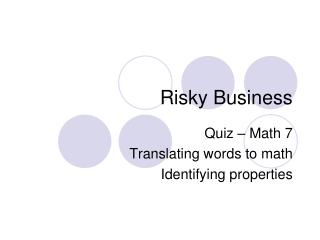DownloadDownload PresentationDownload Presentation- - - - - - - - - - - - - - - - - - - - - - - - - - - E N D - - - - - - - - - - - - - - - - - - - - - - - - - - -
##### Presentation Transcript

1. Risky Business Quiz – Math 7 Translating words to math Identifying properties

2. Question #1 • Write as an algebraic expression • 5 minus a number n

3. Answer #1 • Write as an algebraic expression • 5 minus a number n • 5-n

4. Question #2 • Write as an algebraic expression • 2 less than the product of 10 and a number

5. Answer #2 • 2 less than the product of 10 and a number • 10n - 2

6. Question #3 • Write as an algebraic expression • 63 more than the quotient of a number and 25

7. Answer #3 • 63 more than the quotient of a number and 25 • n/25 + 63

8. Question #4 • Write as an algebraic expression • The product of n and 24, divided by 3

9. Answer #4 • The product of n and 24, divided by 3 • N • 24 ÷ 3

10. Question #5 • Write as an algebraic expression • Triple the sum of a number and 32

11. Answer #5 • Triple the sum of a number and 32 • 3(n + 32)

12. Question #6 • Identify the Property • p(qr) = (pq)r

13. Answer #6 • p(qr) = (pq)r • (APM) – Associative property of multiplication

14. Question #7 • Identify the Property • 1 • 7 = 7

15. Answer #7 • 1 • 7 = 7 • (IPM) – Identity property of multiplication

16. Question #8 • Identify the Property • 0 + 12 = 12

17. Answer #8 • 0 + 12 = 12 • (IPA) – Identity property of addition

18. Question #9 • Identify the Property • 7(a + b) = 7a + 7b

19. Answer #9 • 7(a + b) = 7a + 7b • (DP) – Distributive property

20. Question #10 • Identify the Property • 3 • 0 = 0

21. Answer #10 • 3 • 0 = 0 • (MPZ) – Multiplicative property of zero

22. Question #11 • Identify the property • x+8= 8+x